﻿ 20-sim webhelp > Toolboxes > Mechatronics Toolbox > Servo Motor Editor > Theory > Torque Speed Plot > Losses

# Losses

Navigation:  Toolboxes > Mechatronics Toolbox > Servo Motor Editor > Theory > Torque Speed Plot >

# LossesEvery motor will experience losses, which results in a temperature rise. The maximum allowed temperature is limited by the magnets, the oil in the bearings and the mechanical behaviour of the brushes. Analysis of the cause of losses is therefore important for the correct choice of a motor.

## Electric Dissipation

All motor coils have resistance and will therefore generate heat. The heat flow generated is equal to:which can also be written as:with irms the root mean square current:For constant DC currents the root mean square value is equal to the current itself. For sinusoidal currents, the root mean square value is equal to the amplitude of the current times the square root of 2.

## Hysteresis losses

In all motors except from hollow rotor motors, the magnets move with respect to the iron core of the motor. This results in a reversing magnetic field in the iron which leads to hysteresis.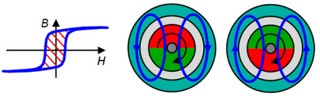Each rotation produces an energy loss proportional to the area of the hysteresis curve (B/H). The power loss due to hysteresis is proportional to the motor speed and can therefore be represented as a friction torque. Due to this friction torque the motor can produce less output torque. This friction torque can therefore be represented in the torque speed plot as a drop down of all curves.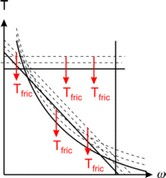## Eddy Current losses

A reversing magnetic field in iron also leads to an induce voltage. The resistance of the iron determines the resulting currents in the iron. These currents are named eddy currents.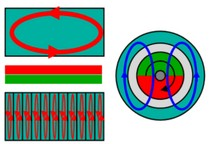Eddy currents heat up the iron because of its resistance. If a massive iron return is used large eddy currents will be induced with high power losses. Therefore in most motors laminated is applied, which reduce eddy currents by decreasing the path length and thus lead to smaller small power losses. The power loss due to eddy currents is proportional to the square of the motor speed and can therefore be modeled as mechanical damping. Damping will decrease the performance of the motor. It can be represented in the torque speed plot as a falling down of curves that increases with the speed.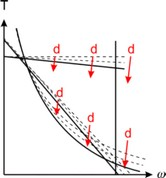## Cogging

In a permanent magnet motors cogging torque manifests itself by the tendency of the rotor to align in a number of stable positions when unexcited. The cogging torque can be described as:where q is the motor angle and p the number of poles. Although cogging does not consume power and is not visible in the torque speed plot, under dynamic conditions it may cause undesirable speed pulsation and also may induce vibrations and acoustic noise.

## Mechanical Losses

Mechanical losses in motors are caused by bearings, brushes and air friction. Only when an air fan is mounted air friction becomes significant. The effects of brushes and bearings can be represented by coulomb friction and damping.

## Temperature

As a result of the heat flow, the motor will warm up leading to two major effects. First of all the coil resistance will increase. Because all coils are made of copper, the temperature dependency of the coil resistance can be written as:where R25 is the coil resistance at room temperature (25 degrees centigrade) and Tcoil the actual coil temperature in degrees centigrade. As a result of the increase of motor temperature, the performance of the magnets will decrease. The result is a decrease of the torque constant :where K25 is the torque constant at room temperature (25 degrees centigrade), Tmagnet the actual magnet temperature in degrees centigrade and ktc the temperature dependency of the used magnet.

 Magnets ktc Ferrites -0.002 Nd2Fe14B -0.0013 SmCo -0.0005 AlNiCo -0.0002

Because the steepness is directly dependent of the torque constant and motor resistancean increase of temperature will lead to a decrease of the steepness. The results of a temperature increase can therefore be shown in the torque speed plot indirectly through the decrease of the maximum torque and steepness.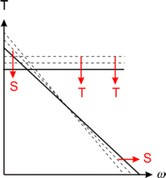## Thermal Duty Cycle

Motor losses result in an increase of the temperature. During short term operation the temperature increase will be limited. During long-term operation, the generated heat and the amount of heat that can be removed via the air and motor mounting determine the temperature increase.

In most motors, the largest portion of the generated heat is cause by electric dissipation. The generated heat is therefore directly proportional to the current and thus the torque. Imagine a motor in long-term operation generating a constant torque. A small torque will lead to only a moderate increase of the motor temperature, but there will be a certain torque where the generated heat leads to an increase of the maximum motor temperature. This is called the maximum continuous torque or the 100% Thermal Duty Cycle (TDC). The line of the maximum continuous torque can be shown in the torque speed plot and is often denoted as T100. For lower speeds the T100 curve will be straight because the heat generation mainly depends on depends on electric dissipation. For higher speeds the T100 curve will decrease because eddy current losses start to become significant.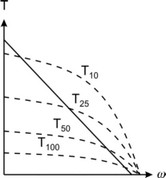If the motor is in continuous operation, but a constant torque is just delivered 50% of the time, a higher torque is permissible. This torque is denoted as T50 and sometimes called the 50% thermal duty cycle. In a similar way T25 and T10 are defined.

## Nominal Operating Point

Many motor manufacturers give in their data sheets a nominal or continuous current at a certain speed. This indicates an operating point on the T100 curve. The nominal or continuous current is the current that continuously be continuously be supplied to the motor at the given speed, which will result in a heating up of the coils to the maximum temperature.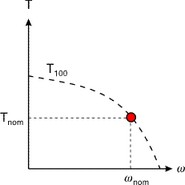The nominal operating point is always based on a certain ambient temperature. In the 20-sim Servo Motor Editor an ambient temperature of 25 ºC is used.

The choice of the speed for the nominal operation point is arbitrary. Some motor suppliers use the speed that results in a maximum power output at the motor shaft. Some motor suppliers use a speed that is half of the maximum allowed speed.